## Cipher## Jun 30, 2017

### Reminder of Sarin gas attack 88 days later - 144/227

http://edition.cnn.com/2017/06/30/middleeast/syria-khan-sheikhoun-chemical-attack-sarin/index.htmlSarin used as weapon in Syria chemical attack = 10+1+9+9+5 + 3+10+5+4 + 1+10 + 5+5+1+7+6+5 + 9+5 + 10+7+9+9+1 + 3+8+5+4+9+3+1+3 + 1+2+2+1+3+2 = 193 (Single Reduction)The attack happened on "April Fourth" = 1+16+18+9+12 + 6+15+21+18+20+8 = 144 (English Ordinal)
In a "rebel-held" town of "Khan Sheikhoun" = 11+8+1+14 + 19+8+5+9+11+8+15+21+14 = 144 (English Ordinal)
rebel-held = 9+5+2+5+3 + 8+5+3+4 = 44 (Full Reduction)
Sarin gas attack = 19+1+18+9+14 + 7+1+19 + 1+20+20+1+3+11 = 144 (English Ordinal)
Chemical weapons attack = 3+8+5+30+9+3+1+20 + 900+5+1+60+50+40+90 + 1+100+100+1+3+10 = 1440 (Jewish)
Forty four = 6+15+18+20+25 + 6+15+21+18 = 144 (English Ordinal)
Assad = 1+19+19+1+4 = 44 (English Ordinal)
44 is often coded with killing and military action - Kill 44 (English Ordinal)Execution = 44 (Full Reduction)Military = 44 (Full Reduction)Genocide = 44 (Full Reduction)....

It's no coincidence that they are talking about this 88 days after the attack
Sarin gas = 19+1+18+9+14 + 7+1+19 = 88 (English Ordinal)
eighty nine killed = 5+9+7+8+2+7 + 5+9+5+5 + 2+9+3+3+5+4 = 88 (Full Reduction)
Poison = 16+15+9+19+15+14 = 88 (English Ordinal)88 days is also 2 months and 27 days, reminding us of 227 (22/7 = 3.14..) - Twenty two divided by seven = 20+23+5+14+20+25 + 20+23+15 + 4+9+22+9+4+5+4 + 2+25 + 19+5+22+5+14 = 314 (English Ordinal)
Sarin gas = 90+1+80+9+40 + 7+1+90 = 318 (Jewish)
7/22 = 0.318... This is a very important number in the bible connecting to the second coming of Jesus Christ, the Sun of God, also the Sun in Greek carries this number
Ηλιος = 8+30+10+70+200 =318GB -> G is the 7th letter, B is the 2nd so we can read this as 72 just like "Syria" = 19+25+18+9+1 = 72 (English Ordinal)

Sarin = 8+26+9+18+13 = 74 (Reverse Ordinal)
poison = 11+12+18+8+12+13 = 74 (Reverse Ordinal)
They are reminding us of this attack 74 days before al-Assad's birthday which is September Eleventh - Assad = 1+90+90+1+4 = 186 (Jewish)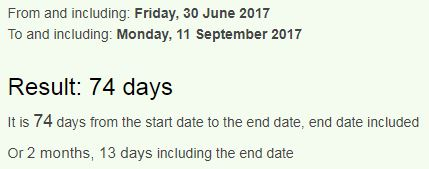If we don't count the end date we see 73 days to his birthday measuring from a date with 73 numerology - 6/30/2017 = 6+30+20+17 = 73
73 is the 21st prime number and Assad's birthday this year lands on a date with 21 numerology (9+1+1+2+0+1+7 = 21)## Jun 28, 2017

### Donald Trump - Emmanuel Macron meeting on Bastille day

They chose a perfect date for the meeting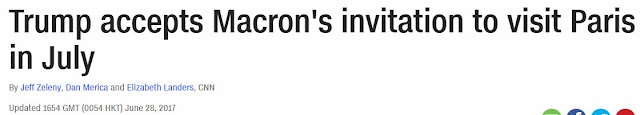\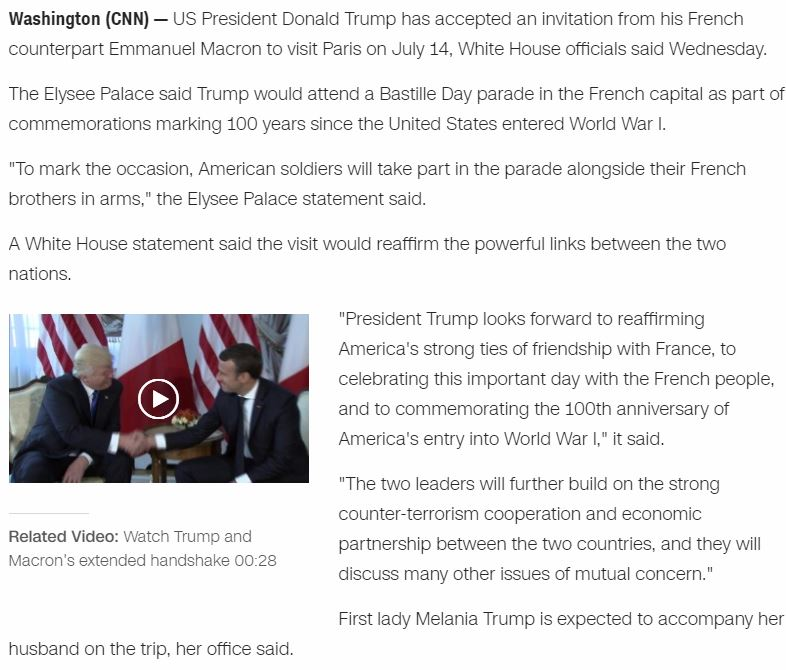The winner of the 58th American presidential election, Mr. Trump = 580 (Jewish) is going to meet French president "Emmanuel Macron" =  58 (Full Reduction) on July 14th, a date with 58 numerology (7+14+20+17), exactly 5 months and 8 days before Macron's birthday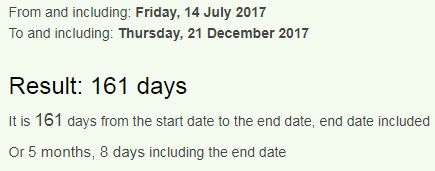Also Trump had his inauguration on a date with 58 numerology - 1/20/2017 = (1+20+20+17)
They are meeting on "Bastille day" = 187 (Reverse Ordinal) in "Paris, France" = 187 (Reverse Ordinal)

Bastille day = 7+8+8+7+9+6+6+4 + 5+8+2 = 70 (Reverse Reduction)
July Fourteenth = 8+6+6+2 + 3+3+6+9+7+4+4+4+7+1 = 70 (Reverse Reduction)They met on 5/25 which is 1 month and 19 days before their next meeting on Bastille day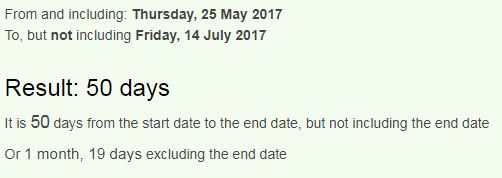Donald = 119 (Jewish), if you've been following the Trump campaign you know how everything connects through 119 and 227 mostly

Here's something even more interesting, Macron and Trump met in Brussels on 5/25 which is 125 days (5x25) since Trump was inaugurated, 125 days is also 17 weeks and 6 days and their next meeting actually comes on 176th day of Trump being in office which happens to be 5 MONTHS and 25 DAYS!Unbelievable...

## Jun 23, 2017

### Spelling of numbers in English language

I made this small discovery about the spelling of numbers a few months ago but I forgot to make a post about it :) We are gonna look at the numbers from 0-9 and you'll see how they should be organized

First I wanna talk about the significance of number 9 for a second
-Any number reduced by it's reflection will always reduce to 9 ....478 - 874 = -396 
-Any number reduced by the sum of it's digits reduces to 9.... 478 - (4+7+8) = 459 
-9 multiplied by any number will always reduce to 9.... 478*9 = 4302 
Also sum of the angles of all regular polygons reduce to 9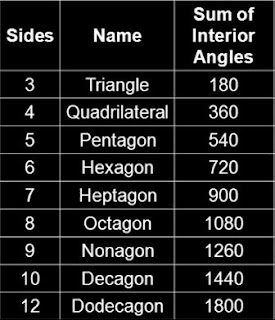When we count to 9 we say 36 (9) letters
one two three four five six seven eight nine = 3+4+4 + 7+4+3 + 7+1+9+4+4 + 3+3+6+9 + 3+9+5+4 + 8+9+3 + 8+4+5+4+4 + 4+9+2+1+7 + 4+9+4+4 = 181 (Reverse Full Reduction)
thirty six letters = 7+19+18+9+7+2 + 8+18+3 + 15+22+7+7+22+9+8 = 181 (Reverse Ordinal)
181 is the 42nd prime number - Nine = 14+9+14+5 = 42 (English Ordinal)

If we take a look at the repeating Fibonacci pattern of 24 digits we find that numbers come in pairs, (1 and 8, 2 and 7, 3 and 6, 4 and 5) all of the pairs adding up to 9

So when we look at the spelling of numbers we just have to put the numbers that are paired next to each other to reveal the secret
-  every pair of numbers that add to 9 will have a total of 8 letters
-  8th triangular number is 36 = 3+6 = 9
Zero + Nine = 8 letters - (0+9 = 9)
One + Eight = 8 letters - (1+8 = 9)
Two + Seven = 8 letters - (2+7 = 9)
Three + Six = 8 letters - (3+6 = 9)
Four + Five = 8 letters - (4+5 = 9)

One Two Three Four Five Six Seven Eight Nine Ten = 6+5+5 + 2+5+6 + 2+8+9+5+5 + 6+6+3+9 + 6+9+4+5 + 10+9+6 + 10+5+4+5+5 + 5+9+7+8+2 + 5+9+5+5 + 2+5+5 = 227 (Single Reduction)
22/7 = 3.14 (Pi), the sum of the first 10 numbers is very fitting considering how we close the circle with 10
Twenty two divided by seven = 20+23+5+14+20+25 + 20+23+15 + 4+9+22+9+4+5+4 + 2+25 + 19+5+22+5+14 = 314 (English Ordinal)

Three hundred sixty = 20+8+18+5+5 + 8+21+14+4+18+5+4 + 19+9+24+20+25 = 227 (English Ordinal)

## Jun 2, 2017

### Saturday Night Live - RKO Movie Set/Rear Window (Murder of Grace Kelly, Princess of Monaco)

The 42nd season finale of Saturday Night Live had some interesting tributes but the one that stood out the most was fart sketch, what's funny about it is the fact that SNL already did a sketch in 2009 where they make exactly the same fart jokes but I can assure you that writers are not running out of ideas, this is most likely connected to the first fart sketch like this called "Rear Window" which is a tribute to the murder of Grace Kelly as you will see after watching these 2 videos.

Grace Kelly died on September 14th, 1982  the day after her car crash. Supposedly she had a stroke behind the wheel and drove off the hill. There's not much conspiracy talk about  her death apart from some reports of her joining Sovereign Order of the Solar Temple just months before her death...
The Rear Window sketch is based on a movie directed by Alfred Hitchcock, the interesting part is how after Kelly's car crash she was dragged out of the vehicle through the 'Rear Window'.

Sir Alfred Joseph Hitchcock = 1+9+9 + 1+3+6+9+5+4 + 1+6+1+5+7+8 + 8+9+2+3+8+3+6+3+2 = 119 (Full Reduction)
Alfred Hitchcock died on April 29th 1980, the 119th day in the non-leap years (4+2+9+1+9+8+0 = 33) also note that he celebrated his last birthday on a date with 119 numerology (8+13+19+79 = 119)Sir Alfred Hitchcock = 8+18+9 + 26+15+21+9+22+23 + 19+18+7+24+19+24+12+24+16 = 314 (Reverse Ordinal), was born in "Leytonstone, Essex" = 30+5+700+200+60+50+100+200+60+50+5 + 5+100+100+5+600 = 2270 (English Extended)Notice the clapboard when they start filming... A. Hitchcock = 1 + 8+9+200+3+8+3+60+3+20 = 315 (English Extended)
"Scene fifteen" = 90+3+5+40+5 + 6+9+6+100+5+5+40 = 314 (Jewish)
Camera three = 3+1+30+5+80+1 + 100+8+80+5+5 = 318 (Jewish)
Both of these sketches end after "take three" = 100+1+10+5 + 100+8+80+5+5 = 314 (Jewish)
Also on the clapboard you see 15 and 1 next to each other which reminds me of 151 that is 227 in octal numbering system (22/7 = 3.14..)

The episode with this sketch came out on the 318th day of the year - 7/22 = 0.318... the opposite of PiNovember Fourteenth = 40+50+700+5+30+2+5+80 + 6+50+200+80+100+5+5+40+100+8 = 1506 (Jewish) - 156th prime number is 911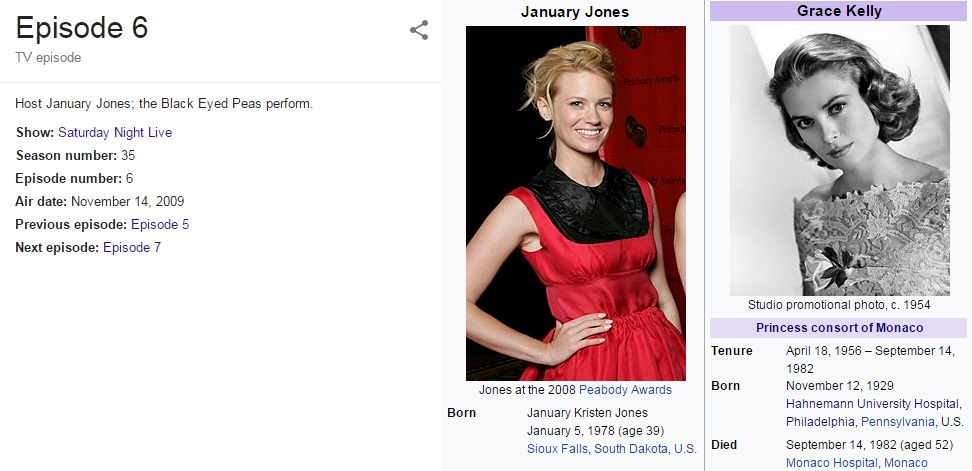In the Rear Window sketch January Jones is playing Grace Kelly on the 314th day from her birthday in 2009January Jones = 17+26+13+6+26+9+2 + 17+12+13+22+8 = 171 (Reverse Ordinal)
Grace Kelly = 20+9+26+24+22 + 16+22+15+15+2 = 171 (Reverse Ordinal)Jason Sudeikis, one of the writers for SNL at the time, is playing James Stewart from the movie Rear Window
Jason Sudeikis = 10+1+19+15+14 + 19+21+4+5+9+11+9+19 = 156 (English Ordinal)
James = 10+1+40+5+100 = 156 (English Extended)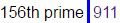At the start of the sketch we see Paramount pictures at the top connecting to 119 once again
Paramount pictures = 60+1+80+1+30+50+200+40+100 + 60+9+3+100+200+80+5+90 = 1109 (Jewish)She died at 10:55 PM after her husband Rainier III, Prince of Monaco took her off life support.
Killing = 20+9+30+30+9+50+7 = 155 (English Extended)

Rainier = 9+8+9+4+9+4+9 = 52 (Reverse Reduction)
It's important to note that she died at the age of 52she was driving back to "Monaco" when she drove off the road, "Monaco" = 4+6+5+1+3+6 = 25 (Full Reduction), they said that stroke is what caused her to lose control, "Stroke" = 1+2+9+6+2+5 = 25 (Full Reduction), "Grace" = 7+9+1+3+5 = 25 (Full Reduction), "Kelly" = 7+4+6+6+2 = 25 (Reverse Reduction), she's born on 11/12/29 = 52
Grace Kelly married Rainier III on 4/18/1956 - 4+18+19+56 =97 (25th prime number)

From her wedding to Prince of Monaco to her death is 26 years, 4 months and 4 weeks..Her death came 4 months and 4 weeks after her 26th wedding anniversary... there's plenty of 44 coding coming up later in the post
twenty six years = 20+23+5+14+20+25 + 19+9+24 + 25+5+1+18+19 = 227 (English Ordinal)
Prince = 70+90+9+50+3+5 = 227 (English Extended)
Twenty two divided by seven = 20+23+5+14+20+25 + 20+23+15 + 4+9+22+9+4+5+4 + 2+25 + 19+5+22+5+14 = 314 (English Ordinal)

Also it's very interesting how Grace Kelly died 3 months and 14 days after Rainier's birthday... look at how nicely π ties into all of this.."Pi" = 16+9 = 25 (English Ordinal), recall how January Jones is playing Grace on the 314th day from her last birthday, also to her next birthday would be 52 days (Grace was 52 years old when she died)automobile accident = 26+6+7+12+14+12+25+18+15+22 + 26+24+24+18+23+22+13+7 = 314 (Reverse Ordinal)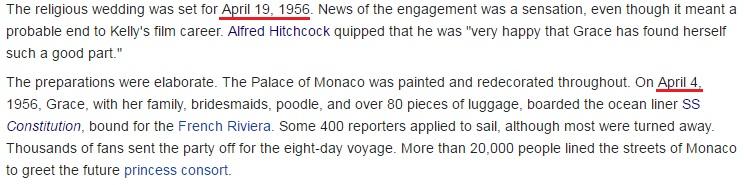Her wedding was originally planned for 4/19/1956 = 4+19 + 1+9+5+6 = 44, also it's important to mention that she set out on her wedding trip on April Fourth  (4/4)...April Fourth = 1+16+18+9+12 + 6+15+21+18+20+8 = 144 (English Ordinal)
The hospital in which Grace Kelly died was later renamed to "Princess Grace Hospital Centre" = 60+80+9+40+3+5+90+90 + 7+80+1+3+5 + 8+50+90+60+9+100+1+20 + 3+5+40+100+5+80 = 1044 (Jewish)
Forty four = 6+15+18+20+25 + 6+15+21+18 = 144 (English Ordinal), Kill = 11+9+12+12 = 44 (English Ordinal), Execution = 5+6+5+3+3+2+9+6+5 = 44 (Full Reduction)

Grace Kelly was born on 11/12/1929 = 11+12+1+9+2+9 = 44, and she died on her 19300th day on Earth after a car crash?! 44th prime number is 193
Car crash = 3+1+9 + 3+9+1+10+8 = 44 (Single Reduction)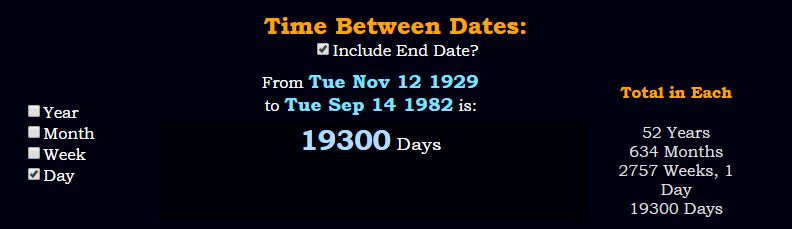It's important to note that Murder by numbers sketch isn't based on anything that RKO Pictures ever produced and they chose it probably because of the connections to 44 and 119 mostly
RKO Pictures was founded on October 14th, 1928 and they released their first movie in the same year she was born, 1929.
10/14/1928 = 10+14+1+9+2+8 = 44, Kelly was also born on a date with 44 numerology
RKO = 18+11+15 44 (English Ordinal)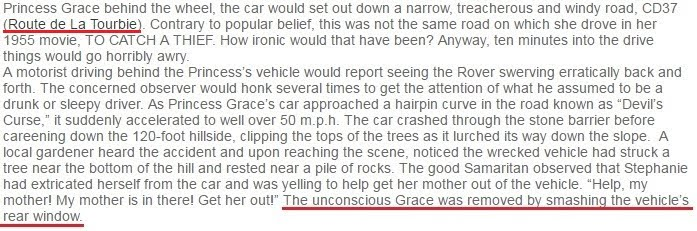Route de la Tourbie = 80+50+200+100+5 + 4+5 + 20+1 + 100+50+200+80+2+9+5 = 911 (Jewish)
To Catch a Thief = 20+15 + 3+1+20+3+8 + 1 + 20+8+9+5+6 = 119 (English Ordinal)
Grace Kelly = 171 (1019 is the 171st prime number)

"Devil's curse" = 4+5+22+9+12 + 19 + 3+21+18+19+5 = 137 (English Ordinal) - the 33rd prime number
Princess Grace = 16+18+9+14+3+5+19+19 + 7+18+1+3+5 = 137 (English Ordinal)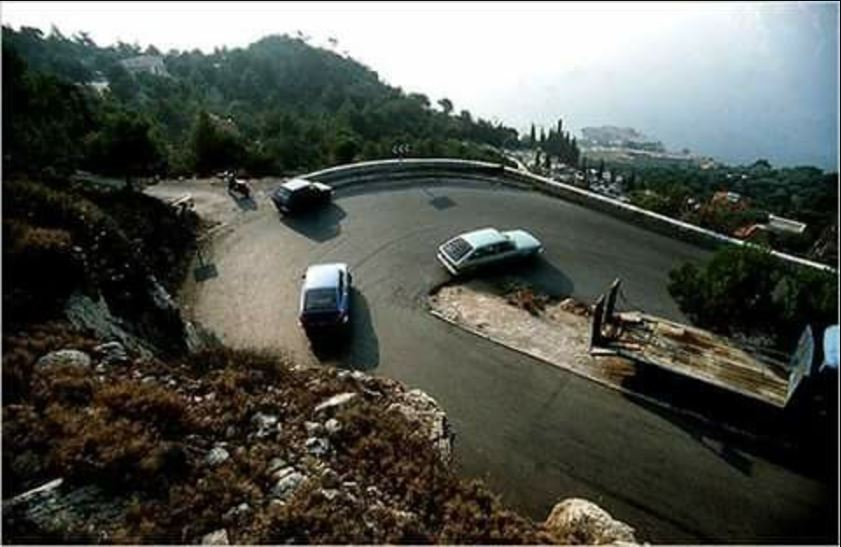Note that Grace Kelly died on 14th of September which connects to 311 - Fourteenth of September = 21+12+6+9+7+22+22+13+7+19 + 12+21 + 8+22+11+7+22+14+25+22+9 = 311 (Reverse Ordinal)Solomon and Klein Detective Agency = 8+3+6+3+5+3+4 + 8+4+5 + 7+6+4+9+4 + 5+4+7+4+6+7+9+5+4 + 8+2+4+4+6+2 = 156 (Reverse Reduction)On the clapboard we see Director: H. Anderson and Producer: K. Sublette
K. Sublette = 11 + 19+21+2+12+5+20+20+5 = 115 (English Ordinal)
Killing = 16+18+15+15+18+13+20 = 115 (Reverse Ordinal)

H. Anderson = 8 + 1+5+4+5+9+1+6+5 = 44 (Full Reduction)

H. Anderson = 8 + 1+40+4+5+80+90+50+40 = 318 (Jewish)
Like in the first sketch they go through 3 takes before the video ends three takes = 100+8+80+5+5 + 100+1+10+5+90 = 404 (Jewish) The video is 4 minutes and 40 seconds longRKO Movie Set = 9+7+3 + 5+3+5+9+4 + 8+4+7 = 64 (Reverse Reduction)

Janet Charmpagne = 1+1+5+5+2 + 3+8+1+9+4+7+1+7+5+5 = 64 (Full Reduction)
Brock Tenderson = 2+9+6+3+2 + 2+5+5+4+5+9+1+6+5 = 64 (Full Reduction)
http://www.vulture.com/2017/05/snl-season-42-finale-recap-dwayne-johnson.html"but that isn't how it was designed." - Is it designed as a follow up tribute of real Murder By Numbers of Grace Kelly? Out of all jokes they could do for this skit they decided to go with copying the Rear Window sketch... How  weird right?

"A few months later, on 13 September, Kelly was out driving with her younger daughter Stephanie when their car crashed over a mountainside, landing, bizarrely, in a garden owned by another Solar Temple member" - this is a quote from one of the links below. If this is in fact true it makes this almost 100% a murder case.
You can read some of the stuff in the links below and decide yourself
http://www.scotsman.com/lifestyle/did-grace-kelly-have-links-with-a-secret-cult-1-474999
http://dev.null.org/psychoceramics/archives/1998.01/msg00027.html
https://stevenhager420.wordpress.com/tag/grace-kelly/
https://savehitchcock.com/2014/05/14/the-truth-why-grace-of-monaco-didnt-play-marnie/